Select Page

# Equation

An equation is an algebraic equality that have a relationship between quantities whose values ​​are unknown and serve to solve problems. However, not all algebraic equalities are equations. An equation expresses, by means of algebraic equality, a relationship between quantities whose value, for the moment, we do not know.

## What is an equation?

The equations are used to code relations in algebraic language and, from there, manage them mathematically. This is a very powerful tool for solving problems.

### How to solve equations

Solving an equation is finding the value, or values, that the letters must take for equality to be true, or finding out that there is no solution. Surely, you know procedures to methodically solve some types of equations. But if we reach the solution by any other way, the resolution is also valid. The solution to the equation is the value of the unknown that makes equality true.

## Examples of equation

Half a number equals one-fifth plus six units:

A number → x
Its half → x / 2
His fifth part → x / 5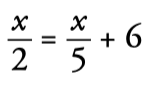Laura’s age coincides with a fifth of the age she will have in 28 years:

Laura’s age → x
Age within 28 years → x +28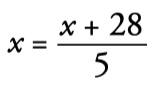A rectangular room is three meters longer than it is wide, and its area is 28 square meters:

Width → x
Length → x + 3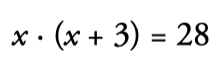## Elements of an equation

#### Members

They are each of the expressions that appear on both sides of the equality.

#### Terms

They are the addends that form the members.

#### Unknowns

They are the letters that appear in the equation.

#### Solutions

They are the values ​​that the letters must take for equality to be fulfilled.

It is the highest of the degrees of the monomials that form the members.

#### Equivalent equations

Two equations are equivalent when they have the same unknowns and the same solutions.

## Equations and identities

• An equation is an equality between algebraic expressions that holds only for certain letter values.
• An identity is an algebraic equality that is always fulfilled, regardless of the values ​​that the letters take.

## Solving equations

### Type x+a=b

How to solve equations type x+a=b:

1. To solve the equation x+a=b, we subtract a from both members.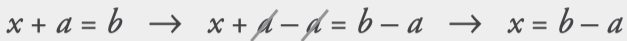2. To solve the equation x+4=7:

We subtract 4 from the two members.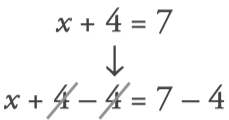3. The solution is x=3: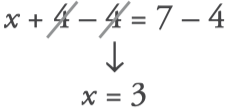### Type x-a=b

How to solve equations type x-a=b:

1. To solve the equation x-a=b, we subtract a from both members.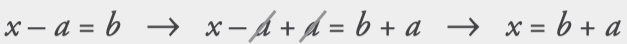2. To solve x-2=6:
We add 2 to the two members: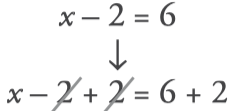3. The solution is x=8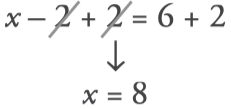## Exercises

1. Calculate the unknown in your mind:

## Ejercicios de ecuaciones de primer grado

2. Apply what you have learned:

## Ejercicios de ecuaciones

### Factors of 26

The number 26 has 4 factors and is a composite number. To calculate the factors of 26 we divide,...

### Factors 25

El número 25 tiene 3 divisores y es compuesto. Para calcularlos dividimos siempre que podamos...

### Factors of 24

The number 24 has 8 factors and is a composite number. To calculate the factors of 24 we divide,...

### Factors of 23

The number 23 has 2 factors and is a prime number. To calculate the factors of 23 we divide,...

### Factors of 22

The number 22 has 4 factors and is a composite number. To calculate the factors of 22 we divide,...

### Factors of 21

The number 21 has 4 factors and is a composite number. To calculate the factors of 21 we divide,...

### Factors of 20

The number 20 has 6 factors and is a composite number. To calculate the factors of 20 we divide,...

### Factors of 19

The number 19 has 2 factors and is a prime number. To calculate the factors of 19 we divide,...

### Factors of 18

Los divisores de 18 son 6 y es compuesto. Para calcularlos dividimos siempre que podamos entre...

### Factors of 17

The number 17 has 2 factors and is a prime number. To calculate the factors of 17 we divide,...